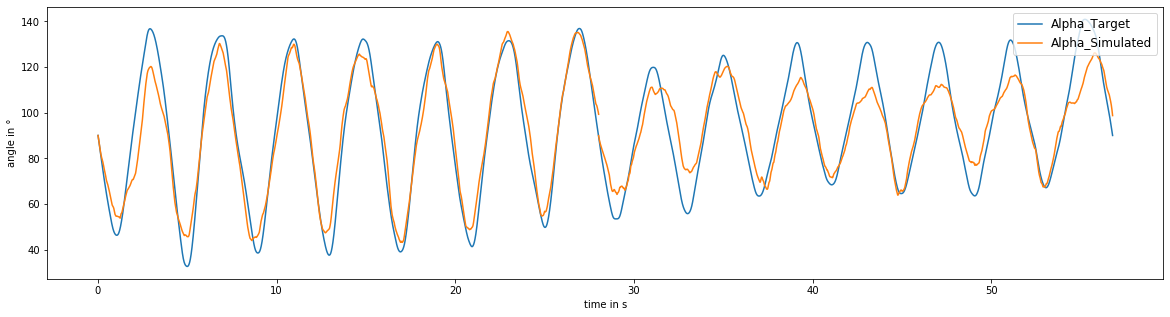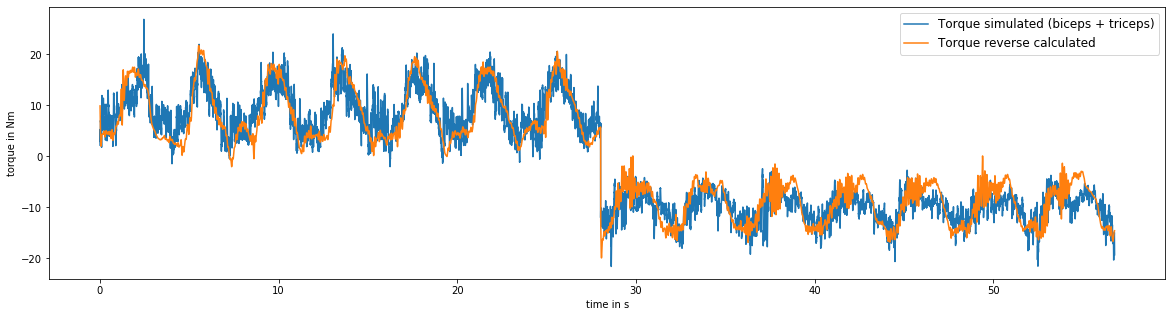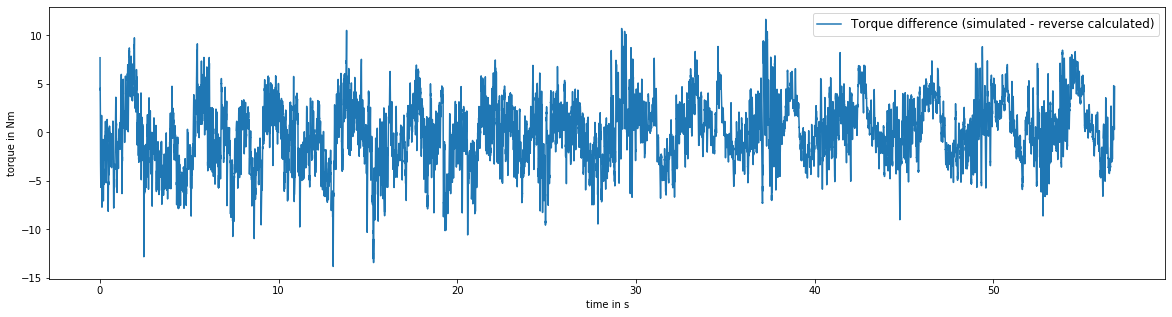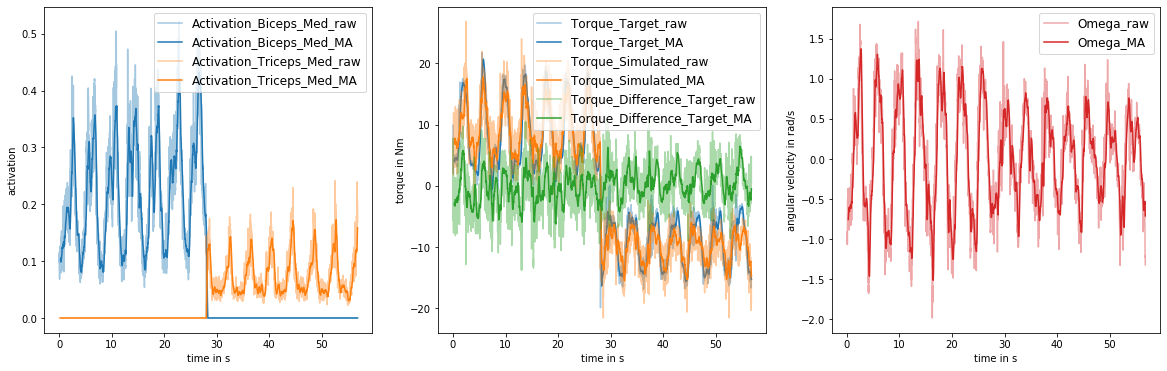torque-difference-predicting-ml-model

# ML-based torque adjustment in hybrid models for limb movement prediction¶

Leserri D. (2020). Based on The use of neural networks in support to existing upper arm models. Unpublished Bachelor module project, Fachhochschule Bielefeld.

This notebook showcases how a multilayer feedforward neural network (MFNN) can be trained to assist an elbow joint model in predicting arm movements. The elbow joint model used here is based on Hill's classic muscle model  and uses electromyography (EMG) signals to predict the angle of an elbow joint. The model has been implemented in MATLAB Simulink. The data used for optimizing the model parameters as well as for training the MFNN is based on experiments in which surface EMG signals of the biceps brachii and triceps brachii -- both muscles which are involved in articulating the elbow -- as well as the correlating joint angle of the elbow where recorded in different subjects.

## Libraries, random seeds and custom functions¶

In this section all necessary python libraries are imported, random seeds are set and custom functions are defined.

In :
# Import of Python libraries
import matplotlib.pyplot as plt, numpy as np, pandas as pd, scipy.io
from sklearn.preprocessing import MinMaxScaler
from sklearn.model_selection import train_test_split
from tensorflow import keras, random as rnd
from IPython.display import display
from warnings import warn

# Set seeds to get reproducable results (not recommended/wanted in general)
np.random.seed(3)
rnd.set_seed(3)

# Custom functions
# A function to calculate the root mean square (RMS)
def rms(data):
return np.sqrt(np.mean(np.square(data)))


## Import data¶

In :
# Load .mat file
filename = 'data/data.mat'

for elem in list(data.keys())[:3]:
del data[elem]

# Format data into correct shape
for elem in data:
data[elem] = data[elem].reshape(len(data[elem]),)

# Convert into Pandas dataframe
data = pd.DataFrame.from_dict(data=data)

# Get column names
columns = list(data.columns)

# Get the index of the first triceps curl exercise and slices of each position
first_triceps_curl_index = data['Position'].value_counts()[-1]
biceps_curls = slice(0, first_triceps_curl_index)
triceps_curls = slice(first_triceps_curl_index, -1)


The provided dataset consists of the following data obtained experimentally:

Key Unit
Activation_Biceps_Med n/a
Activation_Triceps_Med n/a
Alpha_Simulated °
Alpha_Target °
Position n/a
Time s
Torque_Biceps Nm
Torque_Difference_Target Nm
Torque_Simulated Nm
Torque_Target Nm
Torque_Triceps Nm

### Elbow joint model structure¶

Surface electromyography (sEMG) captures the electric potentials of muscle activation, which are translated to muscle activity via electrochemical pathways. This muscle activity is calculated separately for biceps and triceps through activation dynamics  as a first step in the model (cf. figure below). Activation signals range from zero (no activity) to one (maximal activity) and are further translated to forces via simulation of muscle contraction dynamics. A compliant tendon model as well as the joint geometry of the elbow determines emerging torques for each, biceps and triceps. In additon a friction model, which yields negative torque, is implemented to emulate friction of e.g. muscular tissues. Finally a joint angle is calculated based on motion equations. The joint angle and the derived (angular) velocity are fed back recursively within the model.## Deviation of predicted and measured joint angles¶

As shown exemplarily in the figure below, even after optimization of the elbow model parameters, the joint angle over time predicted by the model without assistance of an MFNN deviates from the joint angle actually measured in the subject. In this notebook we assume, that activation dynamics as well as the motion equations in the non-hybrid model are determined in a sufficient precise way. Accordingly, the deviation of the predicted and the measured elbow joint angle is based upon differences in torque estimation by the remaining model compartments.

In :
plt.figure(figsize = (20, 5))
plt.xlabel('time in s')
plt.ylabel('angle in °')
plt.plot(data['Time'].to_numpy(), data['Alpha_Target'].to_numpy(), c = 'C0', label = 'Alpha_Target')
plt.plot(data['Time'].to_numpy(), data['Alpha_Simulated'].to_numpy(), c = 'C1', label = 'Alpha_Simulated')
plt.legend(loc = 1, markerscale = 6, fontsize = 'large');### Torque difference¶

In order to assist the torque estimation of the non-hybrid model using a MFNN the difference in torque prediction of the elbow joint model and the torque actually employed by the subject to move its arm has to be compared. The torque employed by the subject can be reverse calculated. The difference between those torques is assumed to be the known error.

\begin{equation} Torque_{simulated} = T = Torque_{biceps} + Torque_{triceps} \end{equation}\begin{equation} Torque_{difference} = Torque_{reverse\:calculated} - Torque_{simulated} \end{equation}

The associated plots of the predicted and reverse calculated torque as well the resulting torque difference to the previously pictured angle are depicted below.

In :
plt.figure(figsize = (20, 5))
plt.xlabel('time in s')
plt.ylabel('torque in Nm')
plt.plot(data['Time'].to_numpy(), data['Torque_Simulated'].to_numpy(), c = 'C0', label = 'Torque simulated (biceps + triceps)')
plt.plot(data['Time'].to_numpy(), data['Torque_Target'].to_numpy(), c = 'C1', label = 'Torque reverse calculated')
plt.legend(loc = 1, markerscale = 6, fontsize = 'large');In :
plt.figure(figsize = (20, 5))
plt.xlabel('time in s')
plt.ylabel('torque in Nm')
plt.plot(data['Time'].to_numpy(), data['Torque_Difference_Target'].to_numpy(), c = 'C0', label = 'Torque difference (simulated - reverse calculated)')
plt.legend(loc = 1, markerscale = 6, fontsize = 'large');In the following the MFNN (grey box submodel) is trained to predict torque differences based on activation signals, the joint angle and the (angular) velocity. Thus if the MFNN can be trained efficiently based on torque differences, the resulting hybrid model approach can be used to to predict the joint angle more precisely.

## Moving average¶

A comparison between the ML-input signal (muscle activation), the ML-output signal (torque difference) and the final hybrid model output (targeted angle) suggests that key information lies in the lower frequency range (cf. plot below).

Presumably the high frequency characteristics of the activation singnals and torque difference are most likely due to inherited noise from the noisy EMG signal or an internal artifact of the MATLAB Simulink model. Hence it is advisable to apply a low-pass filter to filter superfluous high frequencies.

The moving average operation is a type of convolution with characterics of a low-pass filter and concurrently has the benefit of densing a time window into a single value. Therefore it is well-suited for this type of application, since it is unknown which actual single activation value represents the associated torque difference/angle. A condensed window, depending on its width, represents and includes most likely the actual responsible activation value within a frame of past and/or future activation values.

In this use case moving average is used as a filter with a width of 300 values which represents a time frame of about 333 ms to filter the high frequency characteristics of the activation signals, torque signals and angular velocity.

In :
window_width = 300

ma_data = pd.DataFrame()

for key in data.keys():
if 'Activation' in key or'Torque' in key and not 'Difference' in key or 'Omega' in key:
ma_data[key] = data[key].rolling(window_width).mean()
else:
ma_data[key] = data[key]

ma_data['Torque_Difference_Target'] = ma_data['Torque_Target'] - ma_data['Torque_Simulated']

ma_data = ma_data.dropna()

In :
plot_label = [['Activation_Biceps_Med', 'Activation_Triceps_Med'], ['Torque_Target', 'Torque_Simulated', 'Torque_Difference_Target'], ['Omega']]

y_label = ['activation', 'torque in Nm', 'angular velocity in rad/s']

fig, ax = plt.subplots(1, 3,figsize=(20, 6))
for i in range(3):
for j, key in enumerate(plot_label[i]):
ax[i].plot(data['Time'].to_numpy(), data[key].to_numpy(), label = key + '_raw' , color = 'C' + str(j) if i < 2 else 'C3', alpha = 0.4)
ax[i].plot(ma_data['Time'].to_numpy(), ma_data[key].to_numpy(), label = key + '_MA' , color = 'C' + str(j) if i < 2 else 'C3')
ax[i].set_xlabel('time in s')
ax[i].set_ylabel(y_label[i])
ax[i].legend(markerscale = 6, fontsize = 'large');

/usr/lib/python3/dist-packages/IPython/core/pylabtools.py:132: UserWarning: Creating legend with loc="best" can be slow with large amounts of data.
fig.canvas.print_figure(bytes_io, **kw)## Define input(s) and output(s) of grey box submodel¶

For this specific exemplary hybrid model the inputs and outputs are defined as follows:

Inputs:

• Activation Biceps Medial
• Activation Triceps Medial
• Target Angle
• Angular velocity Omega
• Position

Output:

• Torque Difference
In :
# Specify input and output labels
input_label = ['Activation_Biceps_Med', 'Activation_Triceps_Med', 'Alpha_Target', 'Omega', 'Position']
output_label = ['Torque_Difference_Target']


## Normalizing inputs and outputs¶

For this exemplary ML model the tanh (Hyperbolic Tangent) function is used as the activation function in all layers of the grey box submodel. To achieve a higher convergence of the ML model and operate within the workspace of the tanh, inputs are normalized to a range of -1 to 1.

In :
# Initialize dictionary holding scalers
scaler = {}

for key in np.r_[input_label, output_label]:
# Generating new scaler
scaler[key] = MinMaxScaler(feature_range=(-1, 1))
# Scale data
ma_data[key] = scaler[key].fit_transform(ma_data[key].to_numpy().reshape(len(ma_data[key]), 1))


## Input data¶

A first visual look at the input data is helpful to determine if the chosen dataset is appropriate to represent the given problem and to check if the scaling has been applied correctly. For the experiments subjects were asked to modulate the movement of the lower arm sinusoidally while recording the elbow joint angle and biceps and triceps muscle activation via sEMG. Hence, a periodic modulation of the elbow joint angle ($\alpha$) and the angular velocity ($\omega$) can be observed in the figure depicted below.

In :
fig, ax = plt.subplots(len(input_label), 1, figsize = (20, len(input_label) * 3))

first_triceps_curl_index = ma_data['Position'].value_counts()[-1]
biceps_curls = slice(0, first_triceps_curl_index)
triceps_curls = slice(first_triceps_curl_index, -1)

x_start = ma_data['Time'][ma_data['Time'].index]
x_mid = ma_data['Time'][ma_data['Time'].index[first_triceps_curl_index]]